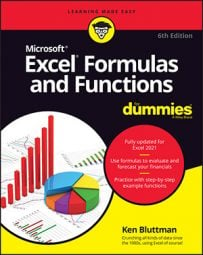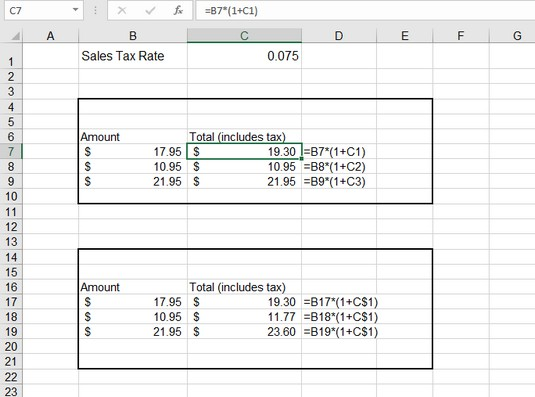##### Excel Formulas & Functions For DummiesThe Excel ADDRESS function takes a row number and a column number as arguments and returns a standard cell reference (cell address). For example, if you pass the row number 4 and the column number 3, the function returns C4. Excel’s ADDRESS function can return an absolute or relative reference in either of Excel's two reference formats. Before you get to the details, here’s a quick review of the differences between absolute and relative cell references:
• A relative reference is expressed as just the column letter and row number (for example, M290). When you copy a formula that contains a relative cell reference, the reference — the row number and the column letter — is adjusted to reflect the location to which you copied the formula.
• An absolute reference has a dollar sign in front of the column letter and the row number (for example, \$M\$290). When you copy a formula that contains an absolute cell reference, the reference does not change.
• A mixed reference has a dollar sign in front of the column letter or the row number (for example, \$M290 or M\$290). When you copy a formula that contains a mixed cell reference, the part of the reference with the dollar sign does not change, but the other part does.
The following image shows a worksheet in which entering a formula with a relative cell reference causes a problem. Totals are the result of adding the tax to the amount. The tax is a percentage (0.075) for a 7.5 percent tax rate. This percentage is in cell C1 and is referenced by the formulas. The first formula that was entered is in cell C7 and looks like this: `=B7*(1 + C1)`.Changing a reference from relative to absolute.

The formula in cell C7 works correctly. It references cell C1 to calculate the total. But if you use the fill handle to copy the formula from cell C7 to cells C8 and C9, there's a problem. The reference to cell C1 changed to cell C2 and C3. Because these cells are empty, the results in cells C8 and C9 are incorrect; they are the same as the amounts to the left. (No tax is added.)

To better understand, column D displays the formulas that are in column C. When the formula in cell C7 was dragged down, the C1 reference changed to C2 in cell C8, and to C3 in cell C9. Often, this is what you want — for Excel to automatically change cell references when a formula is copied. But sometimes, as in this situation, it is not what you want. You need an absolute cell reference.

The formula in cell C17 is almost identical to the one in cell C7 except that the reference to cell C1 has been made row absolute by placing a dollar sign in front of the row number. The formula in cell C17 looks like this: `=B17*(1 + C\$1)`. When this formula was dragged down into C18 and C19, the reference was not adjusted but stayed pointing at cell C1. Note that in this example, only the row part of the reference is made absolute. That's all that is necessary. You could have made the reference completely absolute by doing this: `=B17*(1 + \$C\$1)`. The result would be the same, but it's not required in this example.

Put a dollar sign in front of the column letter of a cell reference to create an absolute column reference. Put a dollar sign in front of the row number to create an absolute row reference.

Excel supports two cell reference styles: the good old A1 style and the R1C1 style. R1C1 style uses a numerical system for both the row and the column, such as this: R4C10. In this example, R4C10 means row 4 column 10.

To change the cell reference style, choose File  →    Options and check the R1C1 reference style in the Working with Formulas area on the Formulas tab. Using the R1C1 format also forces the columns on the worksheet to display as numbers instead of the lettering system. This is useful when you’re working with a large number of columns. For example, column CV positionally is the 100th column. Remembering 100 is easier than remembering CV.

To get back to the Excel ADDRESS function, it takes up to five arguments:

• The row number of the reference
• The column number of the reference
• A number that tells the function how to return the reference. The default is 1, but it can be
• 1 for full absolute
• 2 for absolute row and relative column
• 3 for relative row and absolute column
• 4 for full relative
• A value of 0 or 1 to tell the function which reference style to use:
• 0 uses the R1C1 style.
• 1 (the default if omitted) uses the A1 style.
• A worksheet or external workbook and worksheet reference
Only the first two arguments are required: the row number and column number being addressed. The function returns the specified reference as text.
 Syntax Result Comment `=ADDRESS(5,2)` `\$B\$5` Only the column and row are provided as arguments. The function returns a full absolute address. `=ADDRESS(5,2,1)` `\$B\$5` When a 1 is used for the third argument, a full absolute address is returned. This is the same as leaving out the third argument. `=ADDRESS(5,2,2)` `B\$5` When a 2 is used for the third argument, a mixed reference is returned, with the column relative and the row absolute. `=ADDRESS(5,2,3)` `\$B5` When a 3 is used for the third argument, a mixed reference is returned, with the column absolute and the row relative. `=ADDRESS(5,2,4)` `B5` When a 4 is used for the third argument, a full relative reference is returned. `=ADDRESS(5,2,1,0)` R5C2 When the fourth argument is false, an R1C1-style reference is returned. `=ADDRESS(5,2,3,0)` `RC2` This example tells the function to return a mixed reference in the R1C1 style. `=ADDRESS(5,2,1,,"Sheet4")` `Sheet4!\$B\$5` The fifth argument returns a reference to a worksheet or external workbook. This returns an A1-style reference to cell B5 on Sheet 4. `=ADDRESS(5,2,1,0,"Sheet4")` `Sheet4!R5C2` This returns an R1C1-style reference to B5 on Sheet 4.
Use Excel's ADDRESS function this way:
1. Click a cell where you want the result to appear.
2. Type =ADDRESS( to start the function.
3. Enter a row number, a comma (,), and a column number.

You can also enter references to cells where those values are located.

4. If you want the result to be returned in a mixed or full reference, enter a comma (,) and the appropriate number: 2, 3, or 4.
5. If you want the result to be returned in R1C1 style, enter a comma (,) and enter 0.
6. If you want the result to be a reference to another worksheet, enter a comma and put the name of the worksheet in double quote marks.

If you want the result to be a reference to an external workbook, enter a comma (,) and enter the workbook name and worksheet name together. The workbook name goes in brackets, and the entire reference goes in double quote marks, such as this: `"[Book1]Sheet2"`.

7. Type a ) and press Enter.

Instead of entering a row number and column number directly in ADDRESS, you can enter cell references. However, the values you find in those cells must evaluate to numbers that can be used as a row number and column number.